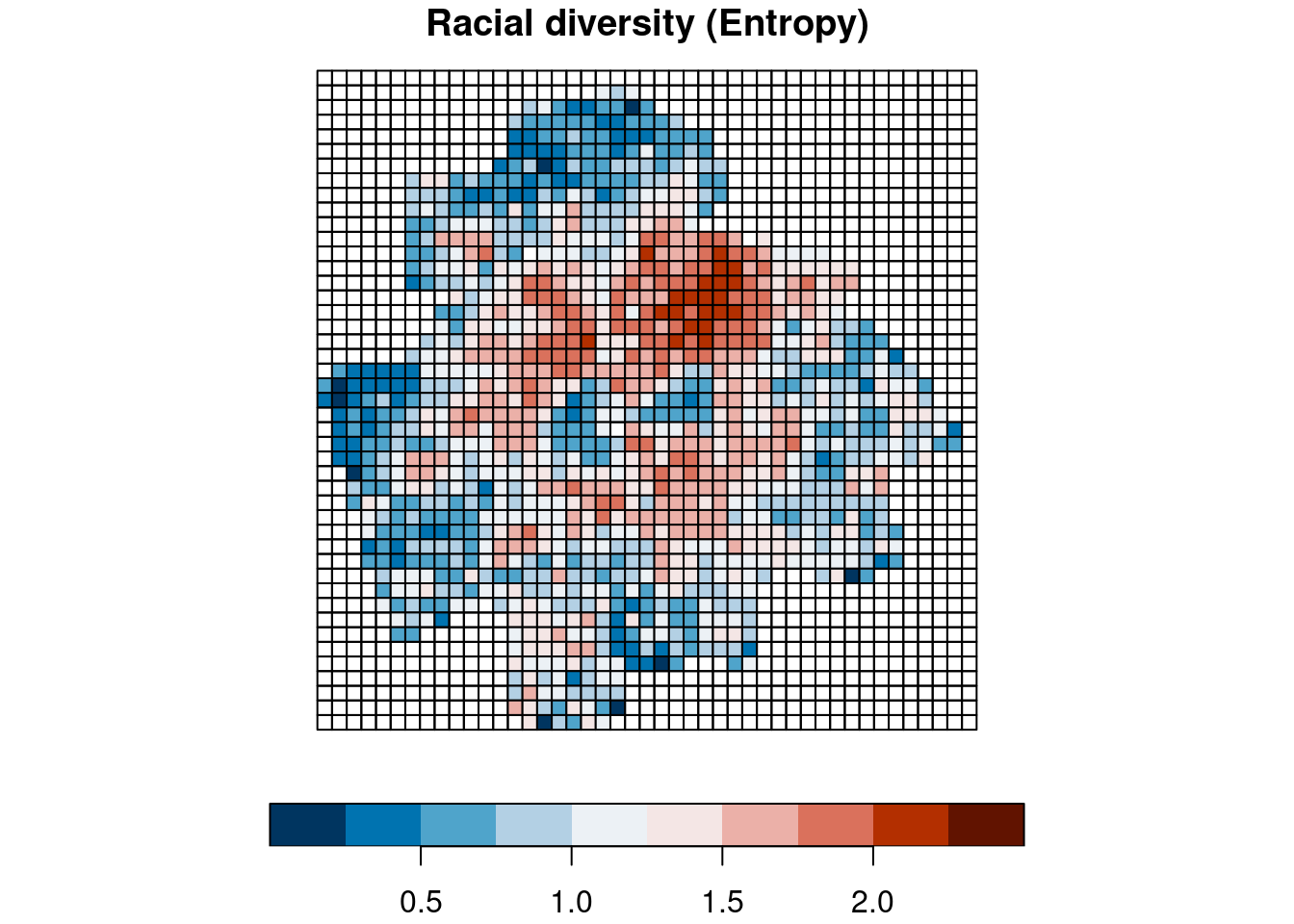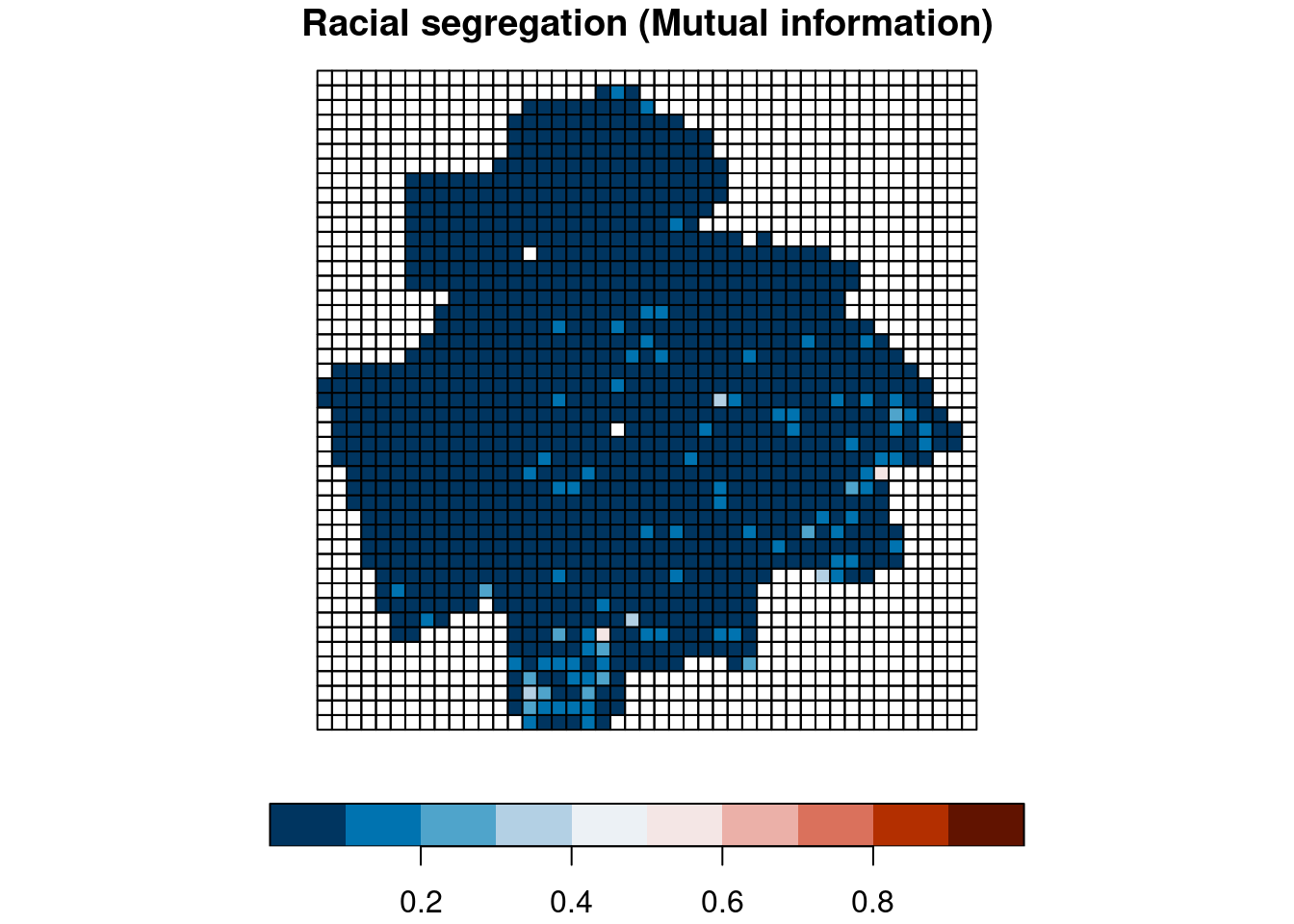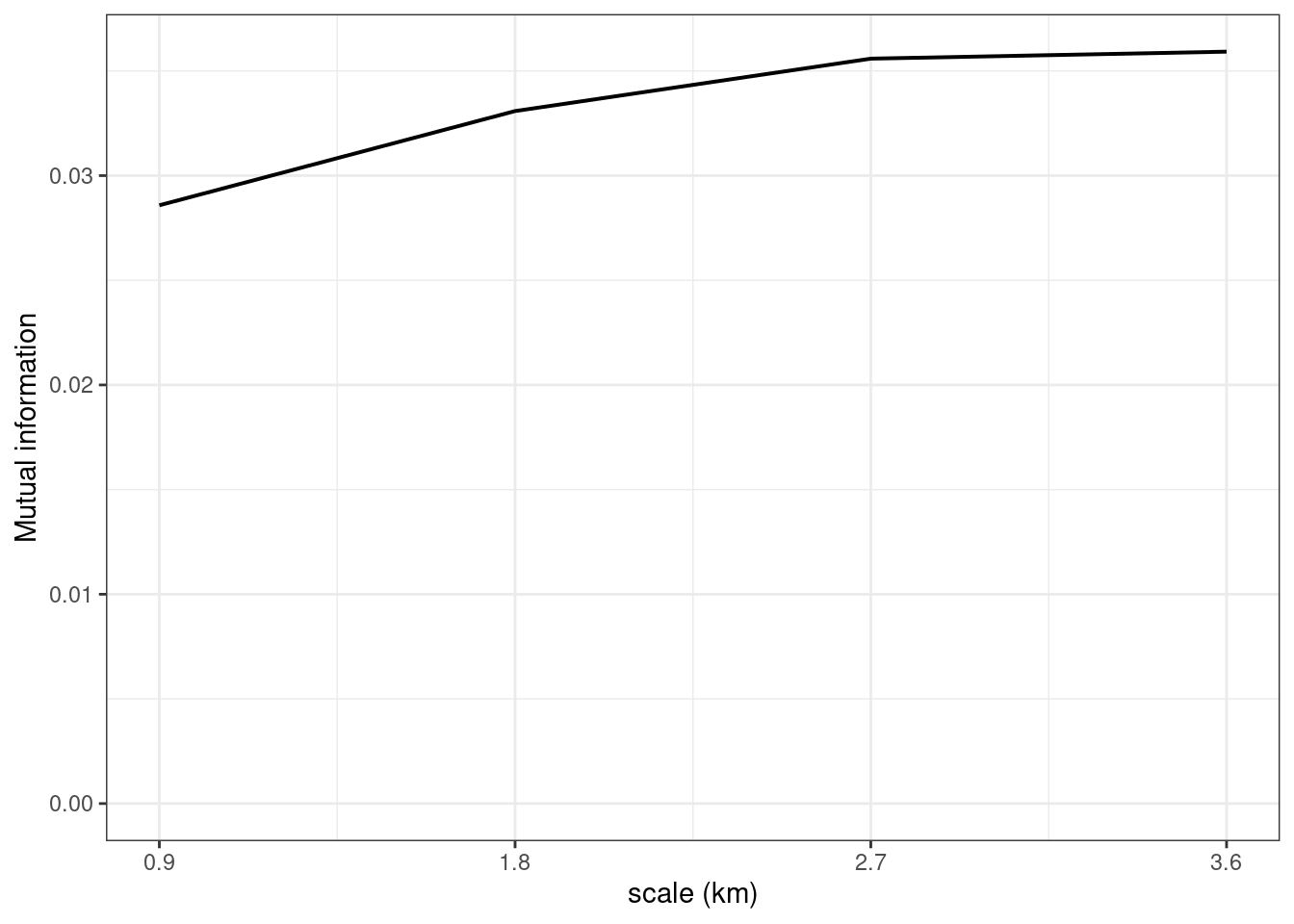This example demonstrates the process of calculating segregation and diversity metrics for the user-defined scale. In the raceland package, scale is defined by the size parameter expressed as a number of cells. RL grids have a resolution of 30 m. Thus the scale will be the multiplication of 30. For example, size = 10 means 10*30m = 300m.

More technical details about calculating racial diversity and segregation metric for a specific scale is included in the raceland tutorial https://cran.r-project.org/web/packages/raceland/vignettes/raceland-intro2.html

# 1 Data

The inputs to calculate racial diversity and segregation metrics for the user-defined scale are RL grid racial ID, and RL grid population density from NRGD2020. This example uses two layers included in the Atlanta dataset. Atlanta dataset can be downloaded from http://www.socscape.edu.pl/index.php?id=nrgd)

• rl_msa.tif - RL grid racial ID cropped to the Atlanta metropolitan statistical area.
• rd_msa.tif - RL grid population density cropped to the Atlanta metropolitan statistical area.

# 2 Calculating metrics for the specific scale

The example below demonstrates how to calculate metrics for the scale of 4.5 km (size = 150). The results are returned as a table showing a value of segregation/diversity in each tile. Additionally, using create_grid() function from the raceland package we can generate spatial object and join the metrics for further visualization.

library(terra)
library(raceland)

#set working directory to the folder containing data using function setwd("")
##setwd("")

#read RL grid racial ID to R using terra package
rl = rast("Atlanta_dataset/RL_grids/rl_msa.tif")
#read RL grid population density to R using terra package
rd = rast("Atlanta_dataset/RL_grids/rd_msa.tif")

#calculate metrics using calculate_metrics from raceland package. Metrics are calculated for each subtile of the specified size.
#Parameter threshold defines how many NA cells can be in the data. In this case, the metrics will be calculated if up to 70% of NA cells are in a given subtile. The metrics are not calculated for subtiles with more than 70% NA cells, and the NA is returned.
metr =  calculate_metrics(rl, rd, neighbourhood = 4, fun = "mean", size = 150, threshold = 0.7)

#metrics can be joined with the spatial object to prepare visualization.
#create_grid from the raceland package creates spatial object
grid_sf = create_grid(rl, size = 150)
attr_grid = dplyr::left_join(grid_sf, metr, by = c("row", "col"))

## 2.1 Visualizing segregation and diversity at the specific scale.

The code below allows to plot racial diversity and segregation map at the specific scale showing the spatial distribution of segregation/diversity within a city.

plot(attr_grid["ent"], breaks = seq(0, 2.6, by = 0.25), key.pos = 1,
pal = rev(hcl.colors(length(seq(0, 2.6, by = 0.25)) - 1, palette = "RdBu")),
bty = "n", main = "Racial diversity (Entropy)")plot(attr_grid["mutinf"], breaks = seq(0, 1, by = 0.1), key.pos = 1,
pal = rev(hcl.colors(length(seq(0, 1, by = 0.1)) - 1, palette = "RdBu")),
bty = "n", main = "Racial segregation (Mutual information)")# Calculating metrics for the range of scales and plotting segregation profile

The code below calculates metrics for a range of user-defined scales. In this example, the scale is defined as 30, 60, 90, and 120 cells, corresponding to the scale of 900m, 1.8km, 2.7km, and 3.6km. The results are saved in the “results” directory created in the working directory. The results directory contains two subdirectories: the shp subdirectory will contain ESRI Shapefiles that can be used for the visualization, the final subdirectory will contain text file metrics.csv with the segregation and diversity indices for each scale, and the segregation_profile.pdf.

library(terra)
library(raceland)
library(sf)
library(ggplot2)

#define scale: size 30 cells -> 900 m, 60 cells -> 1.8km, 90 cells -> 2.7km, 120 cells -> 3.6km
scales = c(30, 60, 90, 120)

#set working directory to the folder containing data using function setwd("")
##setwd("")

#read RL grid racial ID to R using terra package
rl = rast("Atlanta_dataset/RL_grids/rl_msa.tif")
#read RL grid population density to R using terra package
rd = rast("Atlanta_dataset/RL_grids/rd_msa.tif")

# create directory with the results, directory "result" will be created as sub-directory in the working directory
pf = getwd()
dir.create(file.path(pf, "results"), showWarnings = FALSE)
dir.create(file.path(pf, "results", "final"), showWarnings = FALSE)
dir.create(file.path(pf, "results", "shp"), showWarnings = FALSE)

complete_smr_df = data.frame(size = integer())
for (size in scales) {

#######################CALCULATE METRICS FOR SPECIFIED SCALE######################
#calculate metrics using calculate_metrics() from raceland package. Metrics are calculate for each subtile of the specified size.
metr_df = calculate_metrics(rl, rd,
neighbourhood = 4, fun = "mean",
size = size, shift = NULL, threshold = 0.7)
#based on entropy calculate Hill's number
metr_df$hill = 2^metr_df$ent
#calculate NMI - normalized mutual information (mutual information divided by entropy)
metr_df$NMI = metr_df$mutinf/metr_df$ent # Summarize metrics ## number of subtiles at a given scale sel = metr_df[!is.na(metr_df$ent), ]
nsubtiles = nrow(sel)

#Calculate an average value of metrics from all subtiles.
complete_smr = c(scale = (size*30)/1000, n = nsubtiles, E = mean(metr_df$ent, na.rm = TRUE), MI = mean(metr_df$mutinf, na.rm = TRUE), HILL = mean(metr_df$hill, na.rm=TRUE), NMI = mean(metr_df$NMI, na.rm=TRUE))

#complete_smr_df is a data.frame with all metrics calculated for specified scales.
complete_smr_df = rbind(complete_smr_df, complete_smr)

####################### CREATE SPATIAL OBJECT WITH METRICS ######################
# Create spatial object with metrics that will be save as ESRI Shapefile and can be used to show how the segregation/diversity change within a city at the specific scale.
# Create spatial object
grid_sf = create_grid(rl, size = size)

# Join metrics to the grid
attr_grid = dplyr::left_join(grid_sf, metr_df, by = c("row", "col"))
sel_grid = attr_grid[!is.na(attr_grid$ent),] # Save the grid as shapefile st_write(attr_grid, file.path("results", "shp", paste("metr_stat_", size, ".shp", sep = "")), delete_dsn = TRUE) } #stop size loop ## Deleting source results/shp/metr_stat_30.shp' using driver ESRI Shapefile' ## Writing layer metr_stat_30' to data source ## results/shp/metr_stat_30.shp' using driver ESRI Shapefile' ## Writing 49725 features with 9 fields and geometry type Polygon. ## Deleting source results/shp/metr_stat_60.shp' using driver ESRI Shapefile' ## Writing layer metr_stat_60' to data source ## results/shp/metr_stat_60.shp' using driver ESRI Shapefile' ## Writing 12543 features with 9 fields and geometry type Polygon. ## Deleting source results/shp/metr_stat_90.shp' using driver ESRI Shapefile' ## Writing layer metr_stat_90' to data source ## results/shp/metr_stat_90.shp' using driver ESRI Shapefile' ## Writing 5550 features with 9 fields and geometry type Polygon. ## Deleting source results/shp/metr_stat_120.shp' using driver ESRI Shapefile' ## Writing layer metr_stat_120' to data source ## results/shp/metr_stat_120.shp' using driver ESRI Shapefile' ## Writing 3192 features with 9 fields and geometry type Polygon. colnames(complete_smr_df) = c("scale", "n", "E", "MI", "HILL", "NMI") write.csv(complete_smr_df, file.path("results", "final", "metrics.csv")) ####################### CREATE SEGREGATION PROFILE ###################### #plot segregation profile p = ggplot(complete_smr_df, aes(x = scale, y = MI)) + geom_line(linewidth= 0.7) + scale_x_continuous(breaks = complete_smr_df$scale) +
labs(x = "scale (km)", y = "Mutual information") +
ylim(0, max(complete_smr_df\$MI)) +
theme_bw()

p#save segregation profile
ggsave(file.path("results", "final", "segregation_profile.pdf"))